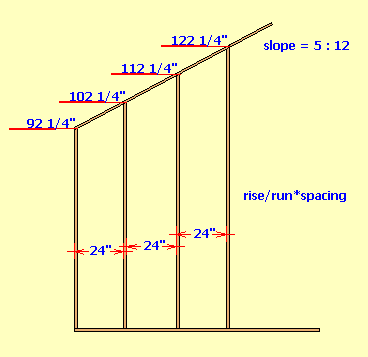# Stud Length Increase - Gable Wall Framing

In a gable (rake) wall the length of the studs will increase by a certain amount based on the spacing of the studs and the slope of the wall.##### Example calculation:
the formula used; rise ÷ run * S, where S = the stud spacing in inches
slope = 5" in 12"
the on-center stud spacing = 24"
`(5 ÷ 12) * 24 = 0.41666666667 * 24 = 10`
The stud length increases by 10 inches.

slope = 2 in 12
the on-center stud spacing = 16".
`(2 ÷ 12) * 16 = 0.1666666667 * 16 = 2.666666666666666667`
Converting the decimal to a fraction by multiplying it by 16 and thus making 16 the denominator
```0.666666666666666667 * 16 = 10.667 rounded to 11
0.666666666666666667 = 11/16```
The stud length increases by 2 11/16 inches

### Gable Wall Stud Length

Slope Ratio /12
Stud Spacing in n
d

#### Results:

 Stud Size Increment in

#### Calculation

1. slope ratio of inches per foot
2. stud spacing in inches

#### Results

1. stud length increase in inches